Reach Us+44-1522-440391
Comparison between Robust and Classical Analysis in Bivariate Logistic for Medical Data | OMICS International
Journal of Biometrics & Biostatistics

Like us on:

All submissions of the EM system will be redirected to Online Manuscript Submission System. Authors are requested to submit articles directly to Online Manuscript Submission System of respective journal.

# Comparison between Robust and Classical Analysis in Bivariate Logistic for Medical Data

Department of Statistics, Al-Furat Al-Awast Technical University, Iraq

*Corresponding Author:
Fadhil Abdul Abbas Al-Abidy
Department of Statistics
Al-Furat Al-Awast Technical University
General Street-Player city, Najaf, Iraq
Tel: +9647801376429
E-mail: [email protected]

Received date: October 06, 2015; Accepted October 26, 2015; Published date: November 02, 2015

Citation: Fadhil Abdul AA (2015) Comparison between Robust and Classical Analysis in Bivariate Logistic for Medical Data. J Biom Biostat 6:262. doi:10.4172/2155-6180.1000262

unrestricted use, distribution, and reproduction in any medium, provided the original author and source are credited.

Visit for more related articles at Journal of Biometrics & Biostatistics

#### Abstract

Representing medical data and biological important part in experiments are concerned with Human life, the primary objective of this research is to use the statistical optimization method analysis for the data and knowledge of the important factors affecting the variables of the study (liver fat, liver size), where the variables are interconnected there is a need for statistical method to examines the degree of their relationship, we used bivariate logistic. To achieve the of the research on the field study will be done in Al-Sadr medical city in the province of Najaf by taking a sample of 150 people auditors diabetes and liver disease center, from the statistical analysis results we observed the degree of diagnosis model in both method are good, and also we monitored that impact factors in responses (liver fat, liver size) and some comment as multivariate logistic in the Future.

#### Keywords

Bivariate logistic; Robust analysis; Logistic regression; Binary regression

#### Aim of Study

This research(study) aims to review the method of bivariate logistic distribution in order to study and analysis effecting factors on the response variables (the degree of liver fatty and increase of liver size in Human beings) using the data of medical tests to compare classical and robust analysis when some values are outlier in the sample.

#### Introduction

In the medical and biological field studies, the experiments are often related to the nature of the response adopted for non- continuous variable data (variables), but is the occurrence/non-occurrence of the score after taking a certain treatment (having effect or non-effect), regardless of the nature of the variables of the study at the left side of the General model equation y=xβ + ε, whether continuous, discrete or categorical.

Where the method of analysis depending on the type of data at the right side (y). If the binary response (0,1 depends on binary logit the method while ordinal response if rank (1st , 2nd , 3rd, ...), for example, the degree of healing of an illness or the degree of incidence of a particular disease is formulated into (generalized) ordinal logit/probit regressions, but if the response takes symbolic letters (A, B, ....) can rely on logit (probit) multinomial

From a historical of view Barry W  studied binary data analysis with bivariate response under the influence of some independent variables and assuming a correlation between paired observations a disturbance depending on the Logistic regression model to estimate parameters the results were obtained are efficient compared with MLE to other researchers.

Kimberlee G, et al.  studied the analysis of correlated binary outcomes using Multivariate Logistic Regression for the case of two outcomes, a form of the cumulative bivariate logistic distribution proposed by Gumbel is used to characterize their joint probabilities in terms of logistic marginal probabilities and the correlation coefficient of the responses. They applied this technique in two different situations. When the correlation among responses is not significantly/and is significantly from zero.

In 1997 Sean M., David B  studied Bayesian analyses of multivariate binary (categorical) outcomes for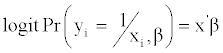where β is a vector of unknown regression coefficients with prior Normal distribution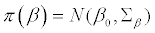Thomas Y  studied Bivariate Binomial Responses by vgam family Functions B= breathlessness, W=wheeze; (B=i, W=j; i, j=0 ,1), but Hun, M. in 2009 studied the Regression Models for binary dependent variables and the analysis data by Using Stata, SAS etc., He used data from clinicians and practitioners simulation study two responses (trust 1 respondent,0 otherwise, www internet used 1 respondent, 0 otherwise) and five independents variables.

In 2014 Tabatabai, MA  and others had studied methods for robust Logistic and probit compared with MLE when there are outlier values, they have been rely on real data and simulation experience for (xi=1,2) as independent variables, they proved robust method is efficient. but they didn't apply bivariate logistic of response.

#### Theory

Logistic distribution

In this part of paper, we display some basic concepts of application distribution Logistic experiments in which the data to the variable appears to stop responding (adopted) is continuous or binary data such as nominal or countable (classified), which does not require a well-known hypotheses linear regression model and there should be no outliers in the data, logistic regression assumes linearity of independent variables and log odds. Only it requires quite large sample sizes, because of maximum likelihood estimate require classification according to the number of response variables (the number of dependent variables) classified to [6,7]:

Binary regression model

This model depends on the following equation: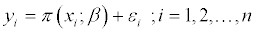Where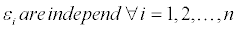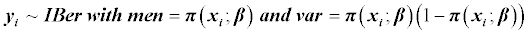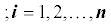a binary response variable (Y=1 or Y=0) is associated a set of explanatory variables, as from the following functions: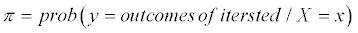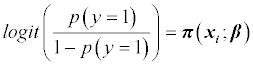is call simple logistic regression

Multiple logistic model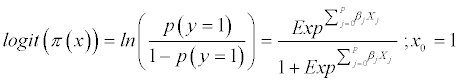After a series of mathematical operations we get the following formula of binary multiple regression: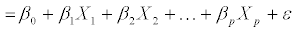We see that the logit of the probability of an event given X is a linear function and then MLE or OLS can be applied to complete inference of parameters β.

Bivariate logistic distribution

This model shows of analysis Logistic in medical studies and biological, for example, eye tests when; one where the response may vary from other response (uncorrelated) or possible interrelated as well as responding in agricultural experiments when two plants in the piece tested one of our show bilateral response, that is,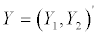and the values of this vectors are as: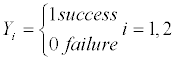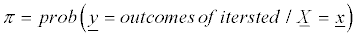We can use bivariate probit regression models and these models have two equations for two binary dependent variables as the following equations: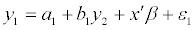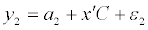Where:

(y1 and y2): are a dummy responses as binary number (0,1), and y2 as endogenous dummy in first equation.

β, C: vectors of parameter's model be estimated.

X: matrix with size (p*n) of independent variables.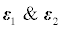errors terms in equations assumed to be independent and identically bivariate standard normal as pdf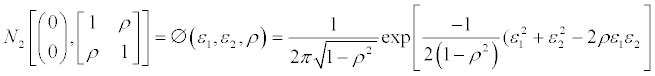and the research relies on statistical estimation methods such as

bivariate OLS or Robust multivariate as M_estimator, R_estimator  but as a result of developments in the software can be relied upon one of these software (Stat) including several functions explains us in the application part [9,10].

#### Statistical Techniques

It was used set of metrics (standards) to determine the statistical model appropriate to the data as well as to test the effect of the independent variables dependent on factors such as: [1,9]

Likelihood-ratio chi-square test statistics or Wald chi-square test and P-values;

Akaike's Information Criteria (AIC) and Bayesian information criteria (BIC);

Parameter estimates and standard errors of the study/exposure variable.

Some tables about spread phenomena (fatty liver, increased liver size) according to Gender and Age classes.

#### Application

Been relying on a random sample data of medical experience in the field of pathological analyzes conducted by researcher  in Al-Sadr Teaching Hospital, the province of Najaf on the study the relationship between blood variables and liver disease(fat & size) on the human where selected a random sample of 150 patients, the study variables:

Dependent variables

Fatty_type - the emergence of the fatty degree on the liver (0 lack of fat,1 the presence of fat).

Liver_type - increase in the Liver size (0 normal, 1 an increase in size).

Independent variables

Age, Gender, Body mass index (BMI), liver enzymes (Gpt, Alk, Got), cholesterol Cho, triglycerides TG, sugar controlled Hb1Ac.

The program was adopted by the statistical software (Stata ver.2014) through analysis Bivariate Binary Logistic Regression, because the response variables in the experiment are binary numbers (1,0), taking into account the lack of independence of the response variables and can be continued to reach the method of implementation through the following illustrative screen  (Figure 1),

Figure 1: Describes lack of independence of the response variables and can be continued to reach the method of implementation through the following Illustrative screen.

The data were analyzed according to the following cases:

Classical method: Do not use Robust analysis we got the following results

Through the results shown in the Table 1 above it is clear to display the following:

Coef. Std. Err z P>lzl (95% Conf. Interval]
Fatty_type
Age -0.0138739 0.0126286 -1 .10 0 .272 -0.038626 0.0108778
Gender 0.0034449 0.2610207 0 . 0 1 0 .989 -0.508146 0.515036
BMI 0.0701801 0.0269094 2. 61 0 .009 0.0174386 0.1229216
HblAc -. 1545435 0.0853633 -1 . 8 1 0 .070 -0.321853 0.0127656
Cho -0.0001859 0.0025515 -o . 0 7 0 .942 -0.005187 0.004815
TG 0.0008252 0.0014075 0 .59 0 . 558 -0.001933 0.0035838
Pressure 0.0074593 0.0076659 0 .97 0 . 3 3 1 -. 0075657 0.0224843
Goe 0.1296733 0.0602018 2 .15 0 .031 . 01168 0.094098
Gpe 0.0184007 0.0386218 0 .48 0. 634 -0.057297 0.0108221
ALI< 0.0019094 0.0045473 0 . 42 0 . 675 -0.007003 0.0108221
cons -2.474073 1.517789 -1. 63 0. 103 -5.448886 0.5007396
Liver_type
Age 0.0249884 0.0125168 2 . 0 0 0 .046 0.0004559 0.0495209
Gender 0.1890935 0.2448802 0 . 77 0 .440 -0.290863 . 669049 9
BMI -0.0045514 0.0225854 -o .20 0 .840 -0.048818 0.0397153
HblAc -0.0882778 0.0824425 -1. 07 0 .284 -0.249862 0.0733065
Cho 0.0070667 0.002851 2 .48 0 .013 0.001479 0.0126545
TG -. 0012979 0.0013507 -o . 9 6 0 . 3 3 7 -0.003945 0.0013493
Pressure 0.0059725 0.00675 0 . 8 8 0 .376 -0.007257 0.0192021
Goe 0.1645504 0.0582545 2 . 8 2 0 .005 0.0503737 0.2787271
Gpe -0.0513203 0.0367339 -1 . 40 0 .162 -0.123318 0.0206769
ALI< -0.0023338 0.0046188 -o .51 0. 613 -0.011386 0.0067188
cons -3 .352374 1.487627 -2 .25 0 .024 -6.268068 -0.4366789
/athrho 0.12393 0.1677924 0 . 74 0 . 460 -0.204937 0.4527971
rho 0.1232994 0.1652415     -0.202115 0.4241955

Table 1: Result analysis of bivariate logistic model by classical method.

The test value (Likelihood Ratio LR) show an appropriate model used for analysis (2=58.34 with P<0.0001) as well as the existence of a positive relationship between the variables of the study accredited

(Fatty_type and Liver_type) (r=0.123), but not significant (p_ value=0.458).

There is effect of some of the independent variables on the dependent variable (Fatty_type) where he showed variable (BMI) very high significant with p_value 0.009, enzyme liver (Got) with probability (p = 0.031) and the degree of a simple effect of sugar control (Hb1Ac) with p_value 0.07, while significant effect of other variables did not show.

The existence of the impact of some independent variables on the dependent variable (Liver_type) where it showed enzyme liver (Got) high impact on increasing the liver size with p_valuep=0.005, fat cholesterol valued likely would (p=0.013) while the age factor is effect with p_value= 0.046, while effect of other variables did not significant.

Robust method: We chosen robust method of analysis to determine the effect of outlier values on the independent variables which assumed some of the values (10%) of them, because the dependent variables are binary data (0,1), the results showed in the following Table 2:

Coef. Std. Err. z P>lzl (95% Conf. Interval]
Fatty_type
Age -0.0138739 0.0135203 -1 .03 0.305 -0.040373 0.0126253
Gender 0.0034449 0.241705 0 .01 0.989 -0.470288 0.477178
BMI 0.0701801 0.0267631 2.62 0.009 0.0177254 0.1226349
HblAc -0.1545435 0.0847402 -1.82 0.068 -0.320631 .0115443
Cho -0.0001859 0.0024457 -o.08 0.939 -0.004979 0.0046076
TG 0.000825 0.001472 0 .56 0 .575 -0.00206 0.00371
Pressure 0.0074593 0.0073579 1 .01 0.311 -0.006962 0.0218805
Goe 0.1296733 . 057284 2 .26 0.024 0.0173988 0.2419479
Gpe 0.0184008 0.0386689 0 .48 0.634 -0.057389 .0941904
ALI< 0.0019094 0.0044956 0 .42 0.671 -0.006902 0.0107207
cons -2.474072 1.703948 -1 .45 0.147 -5.81375 0.8656052
Liver_type
Age 0.0249884 .0116016 2.15 0.031 0.0022496 .0477272
Gender 0.1890935 0.2414576 0.78 0.434 -0.284155 0.6623416
BMI -0.00455 0.023124 -o.20 0.844 -0.04987 0.04077
HblAc -0.0882778 0.0835978 -1 .06 0.291 -0.252127 0.075571
Cho 0.0070667 0.0029229 2 .42 0.016 0.001338 0.0127955
TG -0.0012979 0.0013006 -1 .00 0.318 -0.003847 0.0012511
Pressure 0.0059725 0.007624 0 .78 0 .433 -0.00897 0.0209152
Goe 0.1645504 0.0522388 3. 15 0.002 0.0621643 .2669365
Gpe -0.0513203 0.031757 -1. 62 0 .106 -0.113563 0.0109223
ALI< -0.0023338 0.0041128 -o .570 0 .570 -0.010395 .0057272
cons -3.352374 1.492694 -2 .25 0 .025 -6.278 -0.4267488
/athrho 0.1239301 0.1580029 0 .78 0 .433 -0.18575 0.43361
rho 0.1232995 0.1556008     -0.183643 0.4083338

Table 2: Result analysis of bivariate logistic model by Robust method.

The test value (Likelihood Ratio LR) show an appropriate model used for for analysis (χ2= 71.71 with p <0.0001).

The results in Robust analysis are not different without Robust, for (Fatty_type), where the variable (BMI) has very high effect with p_value==0.009, enzyme liver (Got) with p_value=0.024, but simple effect of sugar control(Hb1Ac) with p_value=0.068, while no significant effect of other independent variables.

The existence of the impact of some of the independent variables on the dependent variable (Liver_type) as variable enzyme liver (Got) high impact on increasing the liver size with p_valuep=0.002 and fat cholesterol is significant with p_value=0.016, while the age factor influential degree p_value=0.031, while significant effect of other variables did not show.

Additional statistical tables are needed to show the numbers of the spread of fatty liver and liver size of the study sample according to social standards (Gender, Age group).

Through the above Table 3 it is clear to us that:

 Gender Fatty Type. Gender Fatty type 0            1 Total 1 18 44 62 2 28 60 88 Total 46 104 150 Age class Liver Type Age class Liver type  0 1 Total 40-55 years 23 42 65 55-70years 19 43 62 <40 years 8 7 15 >70years 1 7 8 Total 51 99 150 Age class Fatty Type Age class Fatty type  01 Total 40-55 years 17 48 65 55-70years 19 43 62 <40 years 5 10 15 >70years 5 3 8 Total 46 104 150

Table 3: Study sample according tosocial standards (Gender, Age group).

The spread of fatty liver in the study sample is 69% (104/150) and the proportion of its spread in females 59% (88/150) compared with males, while increasing the liver size in the research sample that 66% have a problem with increasing the size from the normal, and the highest proportions in the age groups (55-70 years; 40-55 years) 43% and 42% respectively.

While the fat spread problems by 46% in the age group (40- 55 years) and close to this ratio in the category (55-70 years), and is identical with the medical condition after the injury fat in the previous age group starts the problem of increasing the liver size in next age.

#### Conclusion

There is a positive correlation between liver fatty and increase of the size liver responses, but this correlation not significant.

Impact of the explanatory variables (BMI, Age, Hb1Ac, Got, Cho) is approved on liver fatty changes and increased liver size, while do not receive the influence of other explanatory variables on these responses.

Spread of fatty liver disease in females than in males according to the research sample was more prevalent in the age group (40-55 years) while the prevalence of liver size ratio in the age group (55-70 years), a medically acceptable because Category Previous showed the spread of fatty liver disease where after years leads to an increase (inflation) size of the liver.

Recommendations

Use simulations to test the success of the methods used under several influences (errors distribution type, sample size and assuming a high correlation between the two variables of response values).

More studies are required to study the analysis of logistics multivariable models.

#### References

Select your language of interest to view the total content in your interested language

### Article Usage

• Total views: 8418
• [From(publication date):
December-2015 - Sep 22, 2019]
• Breakdown by view type
• HTML page views : 8337

## Post your commentCan't read the image? click here to refresh
###### Peer Reviewed Journals

Make the best use of Scientific Research and information from our 700 + peer reviewed, Open Access Journals

International Conferences 2019-20

Meet Inspiring Speakers and Experts at our 3000+ Global Annual Meetings

Top